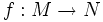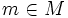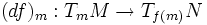# Regular point

Let$f:M \to N$ be a smooth map of differential manifolds. A point$m \in M$ is termed a regular point, or a point of submersion of$f$, if the induced map$(df)_m: T_mM \to T_{f(m)}N$, is surjective.